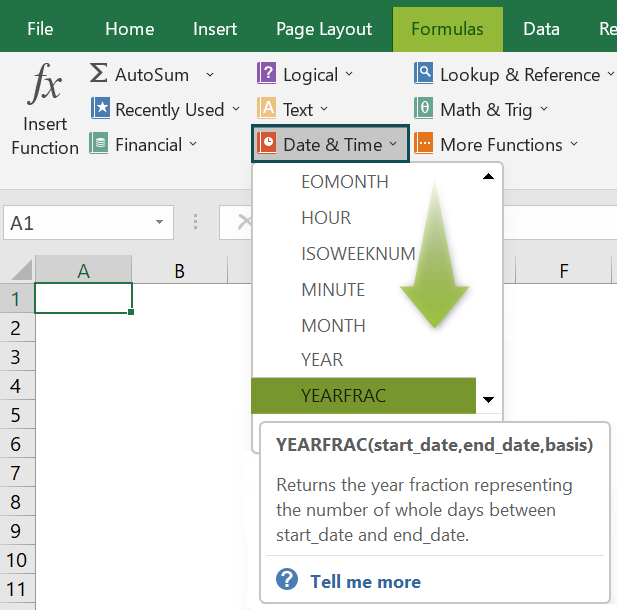# YEARFRAC In ExcelArticle byExcelMojo Team## What Is YEARFRAC function In Excel?

The YEARFRAC Function in Excel calculates the year fraction between 2 dates and returns a decimal value of the fraction.

The YEARFRAC in Excel is an inbuilt “Date & Time” function, so we can insert the formula from the “Function Library” or enter it directly in the worksheet. For example, we will calculate the difference between the two dates using the YEARFRAC().

Select cell C2, enter the formula =YEARFRAC(A2,B2), and press “Enter”. The result is “1”, as shown below.

###### Key Takeaways
• The YEARFRAC in Excel calculates the year fraction between the “start_date” and the “end_date”.
• The dates must be entered in the Excel-recognized format or by using the DATE() function.
• Also, the function will truncate the decimal value provided in the “basis” argument.
• We can find the percentage or proportion of the year using the function.
• We can use the function along with other functions such as YEAR(), TODAY(), DATE(), etc.
• If one of the argument values is not a valid date like non-numeric, text, or alphanumeric, then we will get an error.

### YEARFRAC() Excel Formula

The syntax of the YEARFRAC in Excel Formula is,

The arguments of the YEARFRAC in Excel Formula are,

• start_date: It is a mandatory argument. It is the start date.
• end_date: It is a mandatory argument. It is the end date.
• basis: It is an optional argument. It is the type of day count basis to use.

### How to Use YEARFRAC Excel Function?

We can use the YEARFRAC Excel Function using 2 methods, namely,

1. Access from the Excel ribbon.
2. Enter in the worksheet manually.

#### Method #1 – Access from the Excel ribbon

Choose an empty cell for the output → select the “Formulas” tab → go to the “Function Library” group → click the “Date & Time” option drop-down → select the “YEARFRAC” function, as shown below.

The “Function Arguments” window appears. Enter the arguments in the Start_date”, “End_date”, and Basis fields → click “OK”, as shown below.

#### Method #2 – Enter in the worksheet manually

1. Select an empty cell for the output.
2. Type =YEARFRAC( in the selected cell. [Alternatively, type =Y and double-click the YEARFRAC function from the list of suggestions shown by Excel.]
3. Enter the arguments as cell values or cell references.
4. Close the brackets and press the “Enter” key.

Let us take a basic example to learn this function.

Given the start and end dates, we will calculate the difference between them using YEARFRAC in Excel.

In the table, the data is,

• Column A contains the Start date.
• Here, column B contains the End date.
• Column C displays the Output.

The steps to evaluate the dates by the YEARFRAC in Excel are,

1. Select cell C2, and enter the formula =YEARFRAC(A2, i.e., the starting date.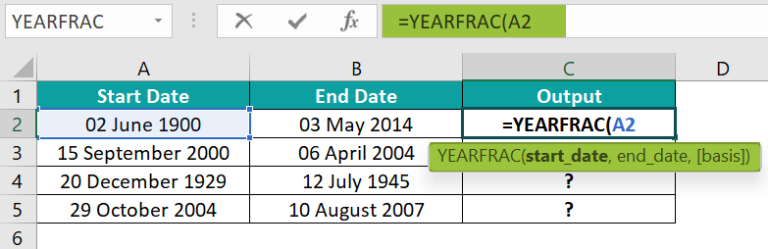2. Enter the “end_date” value as “B2”, i.e., the ending date. The formula is =YEARFRAC(A2,B2,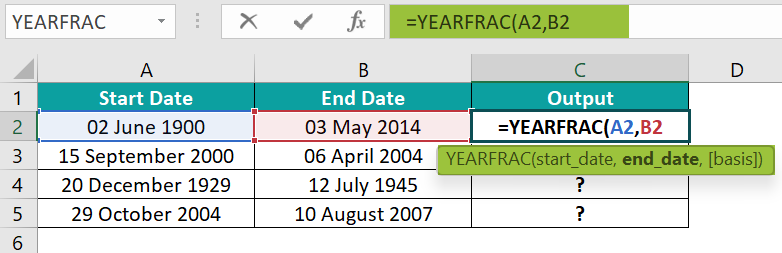3. Enter the value of ‘basis’ as “1”, i.e., the value type, and close the brackets. The complete formula is =YEARFRAC(A2,B2,1)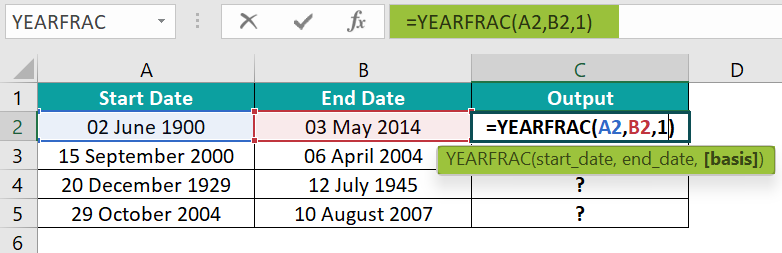4. Press the “Enter” key. The result is “113.919”, as shown below.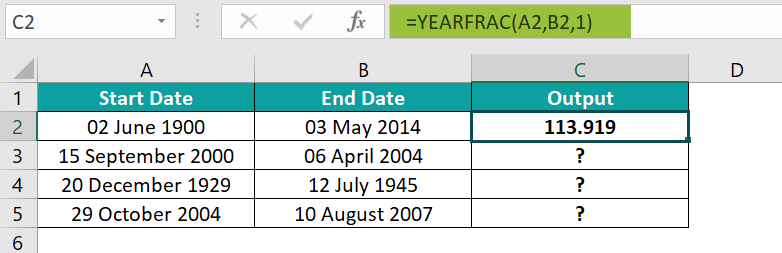5. Drag the formula from cell C2 to C5 using the fill handle. The output is shown below.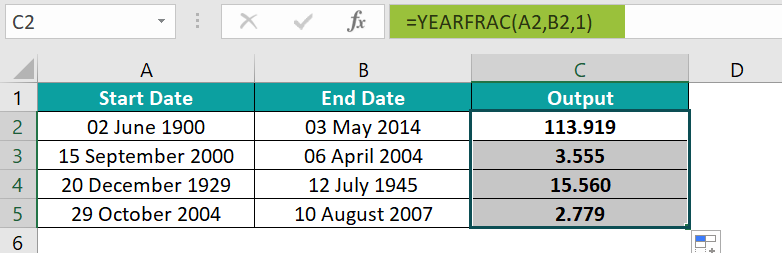### Examples

We will understand some advanced scenarios with the YEARFRAC in Excel examples.

#### Example #1

We will calculate the age using the YEARFRAC() and TODAY Excel functions for the date of birth.

In the table, the data is,

• Column A contains the Name.
• Here, column B contains the DOB.
• Column C displays the Age.

The steps to evaluate the age using the YEARFRAC in Excel are,

• Step 1: Select cell C2, enter the formula =YEARFRAC(B2,TODAY()), and press the “Enter” key.

The result is “8.575”, as shown below.

• Step 2: Drag the formula from cell C2 to C6 using the fill handle in Excel. The output is shown below.

#### Example #2

We will calculate the percentage of the year completed using the YEARFRAC(), YEAR(), and DATE() functions for the dates.

In the table, the data is,

• Column A contains the Date.
• Column B displays the Age.

The steps to evaluate the dates by the YEARFRAC in Excel are,

• Step 1: Select cell B2, enter the formula =YEARFRAC(DATE(YEAR(A2),1,1),A2), and press the “Enter” key. The result is “59%”, as shown below.
• Step 2: Drag the formula from cell B2 to B4 using the fill handle. The output is shown below.

#### Example #3

We will calculate the different errors that occurred using the YEARFRAC for the start date and end date.

In the table, the data is,

• Column A contains the Start date.
• Here, column B contains the End date.
• Column C displays the Output.

The procedure to evaluate the dates by the YEARFRAC in Excel are,

Select cell C2, enter the formula =YEARFRAC(A2,B2), press the “Enter” key, and drag the formula from cell C2 to C5 using the fill handle.

The results in cells C2 to C4 are errors, as shown in the above image. Column D is for our reference.

### Important Things to Note

• The “#VALUE!” error occurs when,
• The “start_date” or the “end_date” values are invalid.
• The “basis” argument is not from the 0 to 4 options.
• We get the “#NUM!” error occurs when the integer portion of the “basis” argument is not between 0 and 4.
• In the formula, if no cell values are present for the selected cells, then we get the result as 0.

1. What does the YEARFRAC in Excel Function do?

The YEARFRAC in Excel calculates the fraction between two dates.

The syntax of the YEARFRAC is =YEARFRAC(start_date,end_date,[basis])

2. How does the YEARFRAC in Excel Function work?

The YEARFRAC in Excel works as follows:

1. Select an empty cell for the output.
2. Type =YEARFRAC( in the selected cell. [Alternatively, type =Y and double-click the YEARFRAC function from the list of suggestions shown by Excel.]
3. Enter the arguments as cell values or cell references.
4. Close the brackets and press the “Enter” key.

For example, the image below depicts the start date and end date, and we will calculate the difference between the dates using the YEARFRAC in Excel.

In the table, the data is,
Column A contains the Start date.
Here, column B contains the End date.
Column C displays the Output.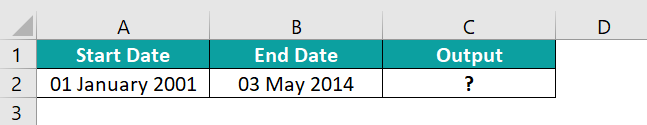The procedure to evaluate the dates by the YEARFRAC in Excel are,

Select cell C2, enter the formula =YEARFRAC(A2,B2), and press the “Enter” key.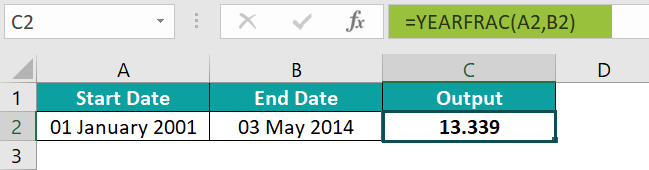3. Where is the YEARFRAC in Excel Function?

The YEARFRAC() is in the “Formulas” tab as follows:

Choose an empty cell for the output → select the “Formulas” tab → go to the “Function Library” group → click the “Date & Time” option drop-down → select the “YEARFRAC” function, as shown below.